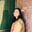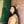Trusted answers to developer questions
Trusted Answers to Developer Questions

Related Tags

c

# What is ilogbl() in C?Umme Ammara

Grokking Modern System Design Interview for Engineers & Managers

Ace your System Design Interview and take your career to the next level. Learn to handle the design of applications like Netflix, Quora, Facebook, Uber, and many more in a 45-min interview. Learn the RESHADED framework for architecting web-scale applications by determining requirements, constraints, and assumptions before diving into a step-by-step design process.

The ilogbl function is a C library function that returns the exponent of a floating point argument as an integer.

The floating point is an alternative way to represent binary numbers with a fractional part.

Every floating point number has three parts:

• Mantissa, which is the actual number.

• Sign, which is the sign (+/-) of the mantissa.

• Exponent, which sets the position of the binary point.

The following illustration shows the parts of a floating point number:

32 bit floating point representation

To use the ilogbl function, we need to include the math.h header file, as shown below:

#include <math.h>


## Syntax

The ilogbl function takes a single argument and returns a single value. It is declared as follows:

## Parameter

The ilogbl function takes a single argument of the long double type.

## Return Value

The ilogbl function returns the exponent of the argument as a signed integer.

• If the argument is zero, the return value is the FP_ILOGB0 macro. The value of FP_ILOGB0 is either INT_MIN or -INT_MAX.

• If the argument is NaN (not-a-number), the return value is FP_ILOGBNAN macro. The value of FP_ILOGBNAN is either INT_MIN or INT_MAX.

• If the argument is infinite, then INT_MAX is returned.

The value of INT_MAX is +2147483647. The value of INT_MIN is -2147483648.

## Syntax

The code below shows the use of the ilogbl function in C:

#include <stdio.h>#include <math.h>int main() {  //Declare values   int exp = 0;   float x  = 2147483644;  //Call the function   exp = ilogbl (x);  //Display the result   printf ("The exponent is: %d\n", exp);  return 0;}

RELATED TAGS

c

CONTRIBUTORUmme Ammara